# A small point mass carrying some positive charge on it, is released from the edge of a table

Question:

A small point mass carrying some positive charge on it, is released from the edge of a table. There is a uniform electric field in this region in the horizontal direction. Which of the following options then correctly describe the trajectory of the mass ? (Curves are drawn schematically and are not to scale).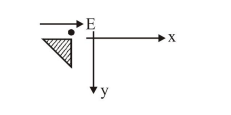1.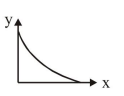2.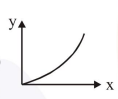3.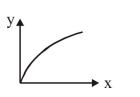4.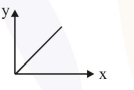Correct Option: 4,

Solution: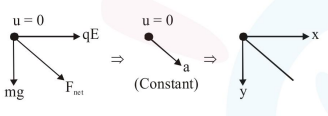Since initial velocity is zero and acceleration of particle will be constant, so particle will travel on a straight line path.